# Problem: A block lies on a plane raised an angle  from the horizontal. Three forces act upon the block:, the force of gravity; , the normal force; and , the force of friction. The coefficient of friction is large enough to prevent the block from sliding (Intro 1 figure).Now you are going to ignore the general rule (actually, a strong suggestion) that you should pick the coordinate system with the most vectors, especially unknown ones, along the coordinate axes. You will find the normal force,, using vertical coordinate system b. In these coordinates you will find the magnitude  appearing in both the x and y equations, each multiplied by a trigonometric function.(A) Because the block is not moving, the sum of the y components of the forces acting on the block must be zero. Find an expression for the sum of the y components of the forces acting on the block, using coordinate system b.Express your answer in terms of some or all of the variables , , , and .(B) Because the block is not moving, the sum of the x components of the forces acting on the block must be zero. Find an expression for the sum of the x components of the forces acting on the block, using coordinate system b.Express your answer in terms of some or all of the variables , , , and .(C) To find the magnitude of the normal force, you must express  in terms of  since  is an unknown. Using the equations you found in the two previous parts, find an expression for  involving  and  but not .

###### FREE Expert Solution

(A)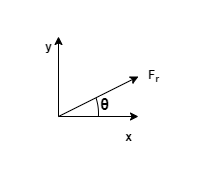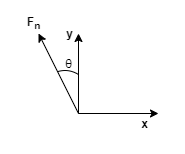For the friction force, θ is measured from the x-axis.

The y-component is equal to Frsinθ directed in the positive y-axis.

For the normal force, θ is measured from the y-axis.

The y-component is equal to Fncosθ directed in the positive y-axis.

Fw is directed in the negative y-axis.

81% (497 ratings)###### Problem Details

A block lies on a plane raised an angle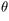from the horizontal. Three forces act upon the block: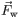, the force of gravity;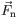, the normal force; and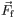, the force of friction. The coefficient of friction is large enough to prevent the block from sliding (Intro 1 figure).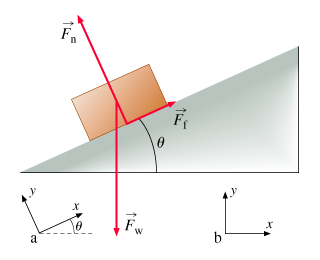Now you are going to ignore the general rule (actually, a strong suggestion) that you should pick the coordinate system with the most vectors, especially unknown ones, along the coordinate axes. You will find the normal force,, using vertical coordinate system b. In these coordinates you will find the magnitude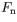appearing in both the x and equations, each multiplied by a trigonometric function.

(A) Because the block is not moving, the sum of the y components of the forces acting on the block must be zero. Find an expression for the sum of the y components of the forces acting on the block, using coordinate system b.

Express your answer in terms of some or all of the variables,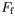,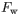, and.

(B) Because the block is not moving, the sum of the x components of the forces acting on the block must be zero. Find an expression for the sum of the x components of the forces acting on the block, using coordinate system b.

Express your answer in terms of some or all of the variables,,, and.

(C) To find the magnitude of the normal force, you must expressin terms ofsinceis an unknown. Using the equations you found in the two previous parts, find an expression forinvolvingandbut not.